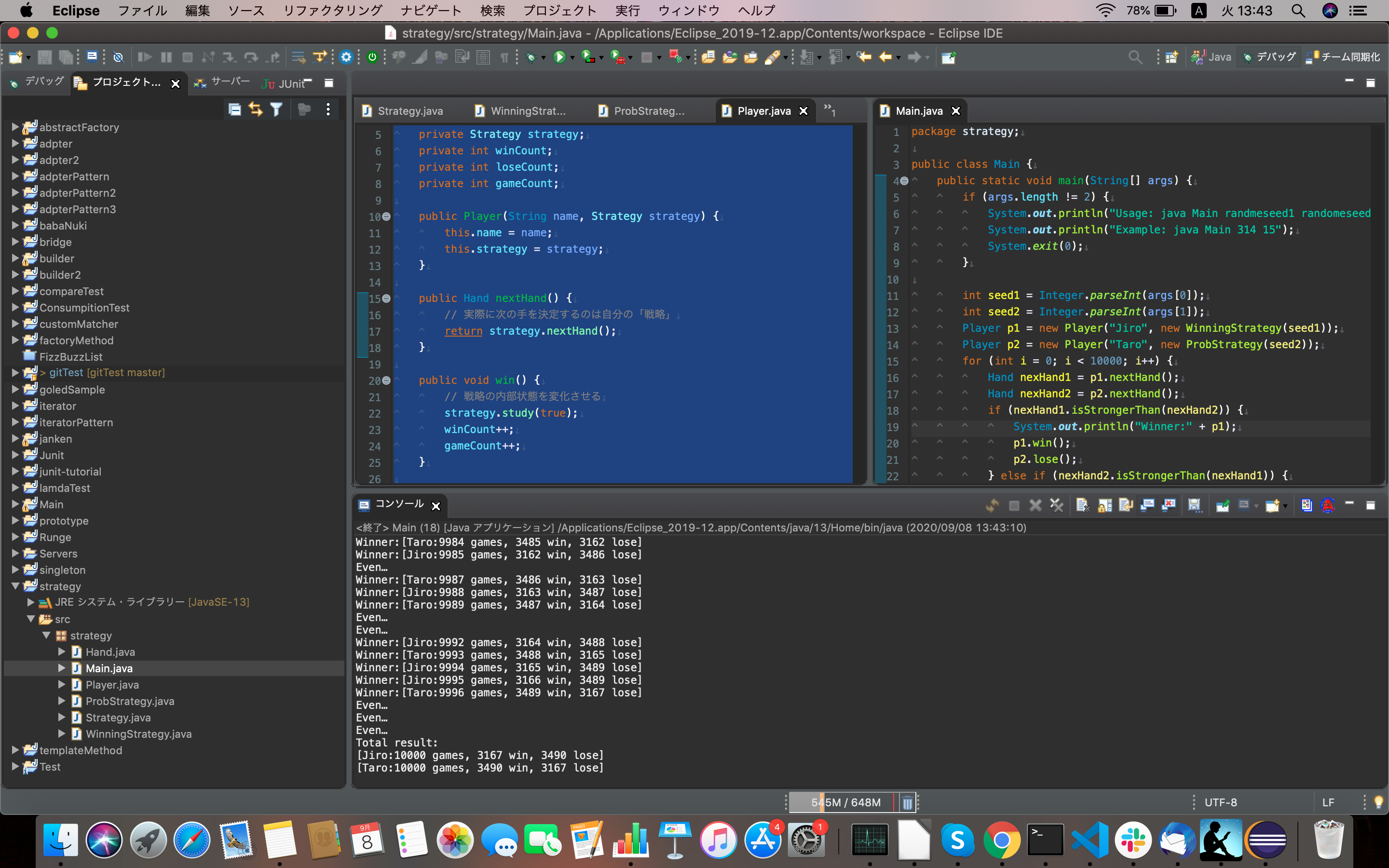# [Java] Strategy pattern

What is the ###Strategy pattern? Any program is written to solve a problem. And a specific algorithm is implemented to solve the problem. In the Strategy pattern, the parts that implement the algorithm can be exchanged. A pattern that makes it easy to switch algorithms and solve the same problem differently.

### Strategy role

The role that defines the interface for using the strategy.

``````package strategy;

public interface Strategy {
// decide next move
public abstract Hand nexHand();
// Learn if you won with your last move
public abstract void study(boolean win);
}
``````

### Role of Concrete Strategy

The role that actually implements the interface of the Strategy role. Here, the strategy (strategy, method, strategy, algorithm) is actually programmed.

``’’ java package strategy;

import java.util.Random;

public class WinningStrategy implements Strategy{ private Random random; private boolean won = false; private Hand prevHand;

public WinningStrategy(int seed) { random = new Random(seed); }

/**

• If you won the last time, put out the same hand, if you lose, randomly decide the hand */ @Override public Hand nextHand () { if (! won) { prevHand = Hand.getHand(random.nextInt(3)); } return prevHand; }

@Override public void study (boolean win) { won = win; } }

``````
```` java
package strategy;

import java.util.Random;

public class ProbStrategy implements Strategy {
private Random random;
private int prevHandValue = 0;
private int currentHandValue = 0;
// A table for probability calculation that reflects past wins and losses
//[The last move] [This time]
private int[][] history = {
{1,1,1,},
{1,1,1,},
{1,1,1,},
};

public ProbStrategy(int seed) {
random = new Random (seed);
}

/**
* Probability is calculated from the value of history
* For example, if the value of history is 3, the value of history is 5, and the value of history is 7.
* The next move is decided by setting the ratio of putting out gou, choki and par to 3:5:7.
* Get a random number from 0 to less than 15 (15 is the sum of 3+5+7),
* If it is 0 or more and less than 3, goo
* 3 or more and less than 8
* Par if 8 or more and less than 15
*
*/
@Override
public Hand nextHand() {
int bet = random.nextInt(getSum(currentHandValue));
int handValue = 0;
if (bet <history [currentHandValue] ) {
handValue = 0;
} else if (bet <history[currentHandValue] + history[currentHandValue]) {
handValue = 1;
} else {
handValue = 2;
}

prevHandValue = currentHandValue;
currentHandValue = handValue;
return Hand.getHand (handValue);
}

private int getSum(int hv) {
int sum = 0;
for (int i = 0; i <3; i ++) {
sum += history[hv][i];
}
return sum;
}

/**
* Update the contents of the history field based on the outcome of the hand returned by the nextHand method.
*
*/
@Override
public void study(boolean win) {
if (win) {
history[prevHandValue][currentHandValue]++;
} else {
history[prevHandValue][(currentHandValue + 1) %3]++;
history[prevHandValue][(currentHandValue + 2) %3]++;
}
}
}
``````

### Context role

A role that uses the Strategy role. It has an instance of ConcreateStrategy role and uses it as needed. It is the interface that plays the role of Strategy.

``’’ java package strategy;

public class Player { private String name; private Strategy strategy; private int winCount; private int loseCount; private int gameCount;

public Player(String name, Strategy strategy) { this.name = name; this.strategy = strategy; }

public Hand nextHand () { // It is your “strategy” that actually decides your next move return strategy.nextHand(); }

public void win() { // change the internal state of the strategy strategy.study(true); winCount++; gameCount++; }

public void lose() { // change the internal state of the strategy strategy.study(false); loseCount++; gameCount++; }

public void even() { gameCount++; }

public String toString() { return “[” + name + “:” + gameCount + “games, “+ winCount + “win, “+ loseCount + “lose” + “]”; } }

``````
### Caller

``'' java
package strategy;

public class Main {
public static void main (String [] args) {
if (args.length != 2) {
System.out.println("Usage: java Main randmeseed1 randomeseed2");
System.out.println ("Example: java Main 314 15");
System.exit(0);
}

int seed1 = Integer.parseInt(args);
int seed2 = Integer.parseInt(args);
Player p1 = new Player("Jiro", new WinningStrategy(seed1));
Player p2 = new Player("Taro", new ProbStrategy(seed2));
for (int i = 0; i <10000; i++) {
Hand nexHand1 = p1.nextHand();
Hand nexHand2 = p2.nextHand();
if (nexHand1.isStrongerThan(nexHand2)) (
System.out.println("Winner:" + p1);
p1.win();
p2.lose();
} else if (nexHand2.isStrongerThan (nexHand1)) {
System.out.println ("Winner:" + p2);
p1.lose ();
p2.win();
} else {
System.out.println ("Even…");
p1.even ();
p2.even();
}
}

System.out.println("Total result:");
System.out.println (p1);
System.out.println(p2);

}
}
``````

### Execution resulthttps://github.com/aki0207/strategy

Tags:

Updated: